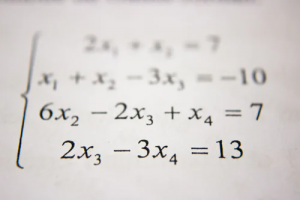# Most Common SAT Math Concepts

When I earned a perfect score on the SAT, it unlocked a world of opportunities and possibilities for me. While it is certainly difficult to ace the SAT, it is entirely possible with the right resources and preparation.

One of the main obstacles that stand in the way of many students’ dream score is the SAT Math section.You can make sure you do well on this section of the test by preparing for the different math concepts that this section covers:

• Heart of Algebra
• Problem Solving and Data Analysis

Here is a breakdown of these common SAT Math concepts that you will see on the test:

## 1. Heart of Algebra questions

Heart of Algebra questions will require you to analyze and solve linear equations, solve problems using the relationship between linear equations and inequalities, and create linear equations. You will also encounter questions related to solving and analyzing functions and interpreting data from graphs.

Here are the topics that fall under this category:

• Solving linear equations and linear inequalities
• Interpreting linear functions
• Solving linear inequality word problems
• Solving linear equation systems
• Graphing linear equations
• Solving linear equation word problems

You might run into a question like this:

Which of the following numbers is NOT a solution of the inequality 6x-5>4x-7?

A. 1
B. 0
C. -1
D. -2

The answer, which is B by the way, requires you to solve a system of linear equations.

## 2. Passport to Advanced Math questions

Unlike Heart of Algebra questions, the Passport to Advanced Math questions on the SAT Match section deal with nonlinear expressions.

Under this category, you can expect to see questions about the following topics:

• Analyzing structure with expressions
• Analyzing rational exponents
• Exponential word problems
• Solving nonlinear equations
• Interpreting nonlinear functions
• Interpreting data from nonlinear graphs

For example, you might see a question that provides a function and then asks the following:

For the function h above, where g is a constant, and h(4) =24 what is the value of h(-6)?

## 3. Problem Solving and Data Analysis questions

For the Problem Solving and Data Analysis questions on the SAT Math section, you will want to brush up on your knowledge of ratios and percentages. You will also want to practice looking at visual elements like charts, tables, and graphs.

Here are the topics that fall into this category:

• Creating ratios, rates, and proportions
• Analyzing tables, scatterplots, graphs, and charts
• Tracking linear and exponential growth
• Analyzing distributions
• Data collection and interpretation

You might be asked questions about calculating percentages based on data from a table or a graph, for example.

## 4. Additional Topics in Math

The Additional Topics in Math questions only comprise a small percentage of the SAT Math section, but you will still need to practice them in order to do well on the SAT. For questions in this category, you will need to understand geometry and trigonometry as well as complex numbers.

Here are some of the topics that are covered by the questions in this category:

• Determining the volume and area of a shape
• Calculating angles of right triangles
• Solving problems using trigonometry
• Analyzing trig functions
• Understanding congruence and similarity

For this part of the SAT Math section, you might see questions asking you to solve a right triangle problem using a trigonometric function, for instance.

## Preparing for the SAT Math section

The best way to make sure you are comfortable with all of the topics that will be covered in the SAT Math section is to take several practice tests and learn key SAT Math strategies.